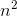# Computer Science : Comparing Run Times

## Example Questions

### Example Question #14 : Algorithm Analysis

for( int i = 0; i < n; ++i){

for( int j = 1; j < n; j *= 2){

someFunction();

}

}

For the code above, what is the run time in Big O notation?

None of the above

O(n log(n) )

O()

O( n )

O( log(n) )

At first glance we might be tempted to pick O() because there are 2 for loops. But, upon closer inspection we can see that the first loop will yield a O( n ) running time but the second loop does not. The second loop has only an O( log(n) ) running time because "j" doubles each iteration and does not increase linearly. That will yield O( log(n) ) since O( log(n) ) is a much faster running time. So the final result is O( n log(n) ).